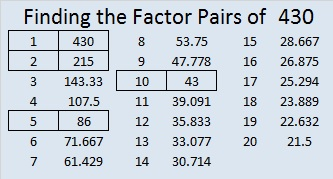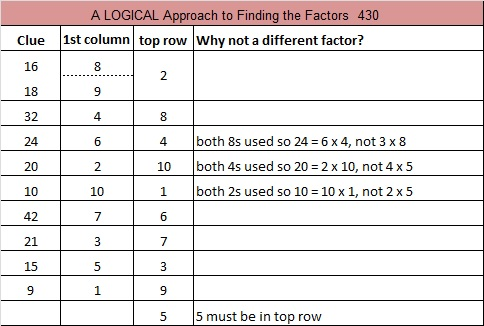# 430 and Level 4

The factors of 430 are listed after the puzzle.

What interesting fact could I say about the number 430? I did a little research.

Biblewheel.com quotes a verse from Galatians 3:17 in the Bible: “And this I say, that the covenant, that was confirmed before of God in Christ, the law, which was four hundred and thirty years after, cannot disannul, that it should make the promise of none effect.” Biblewheel states that 430 means the law, just as 666 means the beast.

OEIS.org says that 430 “is the number of necklaces possible with 6 beads, each being one of 4 colors.” If I understood what that means in English, I’m sure I could construct it mathematically. I know it can’t mean 6 Choose 4. If you understand what it means, please comment.Print the puzzles or type the factors on this excel file:10 Factors 2015-03-16

• 430 is a composite number.
• Prime factorization: 430 = 2 x 5 x 43
• The exponents in the prime factorization are 1, 1, and 1. Adding one to each and multiplying we get (1 + 1)(1 + 1)(1 + 1) = 2 x 2 x 2 = 8. Therefore 430 has exactly 8 factors.
• Factors of 430: 1, 2, 5, 10, 43, 86, 215, 430
• Factor pairs: 430 = 1 x 430, 2 x 215, 5 x 86, or 10 x 43
• 430 has no square factors that allow its square root to be simplified. √430 ≈ 20.7364